# Lakhmir Singh Solutions Class 9 Physics Chapter 3 Gravitation

We know that the earth attracts every object towards itself with the help of the force of attraction which is known as the gravitation or gravitational pull.

According to the universal law of gravitation, every object in the universe attracts every other object by the force of attraction which is directly proportional to the product of masses of the objects and is inversely proportional to the square of the distance between them. This is mathematically given as:

 F = G(mM/d2)

Where,

• F is the force of the gravitational pull
• G is the constant known as gravitational constant
• M is the mass of the object 1
• m is the mass of the object 2
• d is the distance between object 1 and object 2

The universal law of gravitation is responsible for the motion of all the planets around the sun, responsible for the motion of the moon around the earth. It can be said that the law of gravitation binds us to the earth.

When an object falls from a certain height which is under the influence of gravitational force, it is known as the free fall. The speed with which the object falls towards the ground is dependent on the acceleration due to gravity which is denoted by the g and is equal to 9.8 m/s2. The unit if g is m/s2. There are two factors that affect the value of g and they are:

• The radius of the earth. As the radius of the earth increase from the poles towards the equator, the value of g becomes greater at the poles than at the equator.
• As the hight increases, the value of g decreases.

It is important to understand that mass and weight also play an important role. The mass is defined as the quantity of matter that is contained in a body while weight is defined as the force with which an object gets attracted towards the centre of the earth.

## Download PDF Of Lakhmir Singh Physics Class 9 Solutions For Chapter 3 Gravitation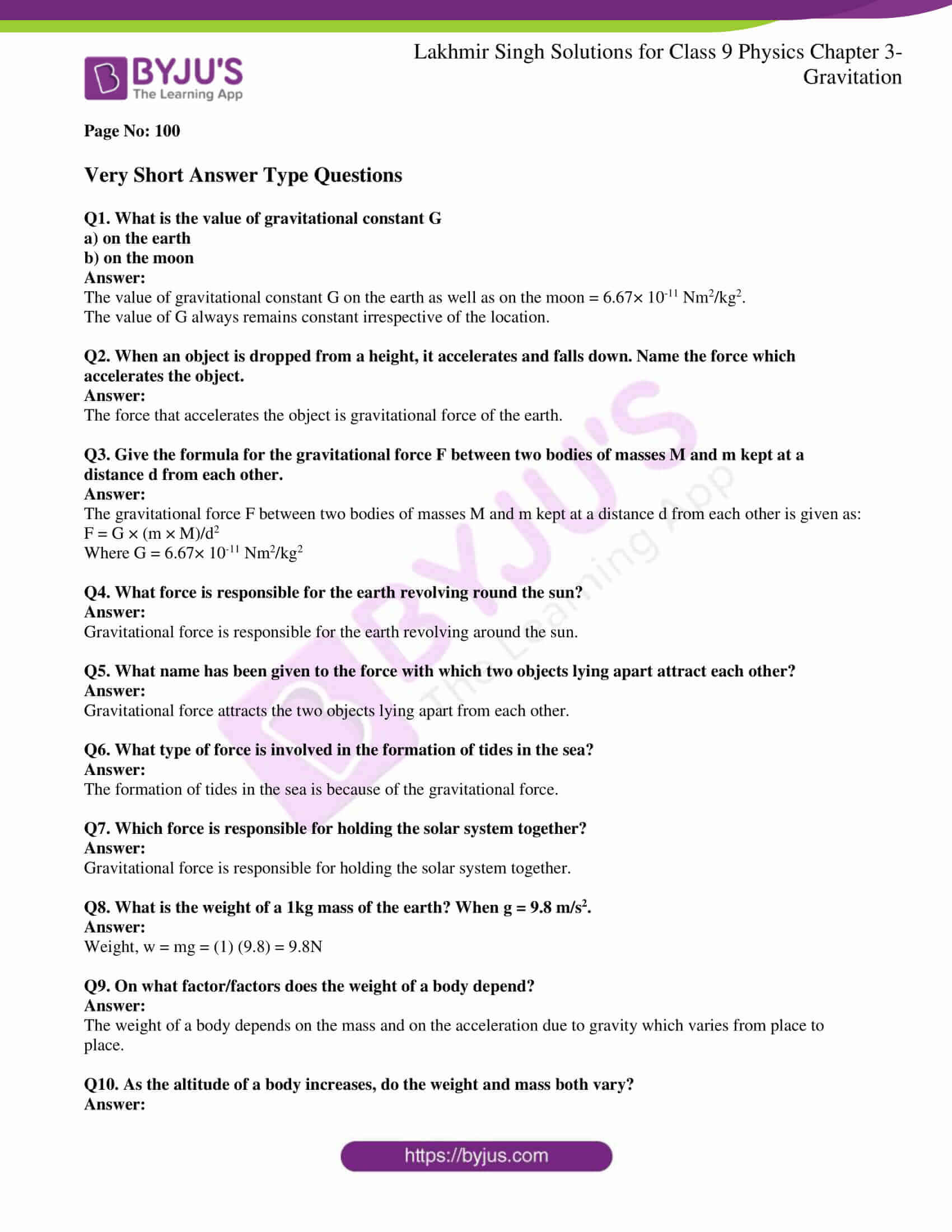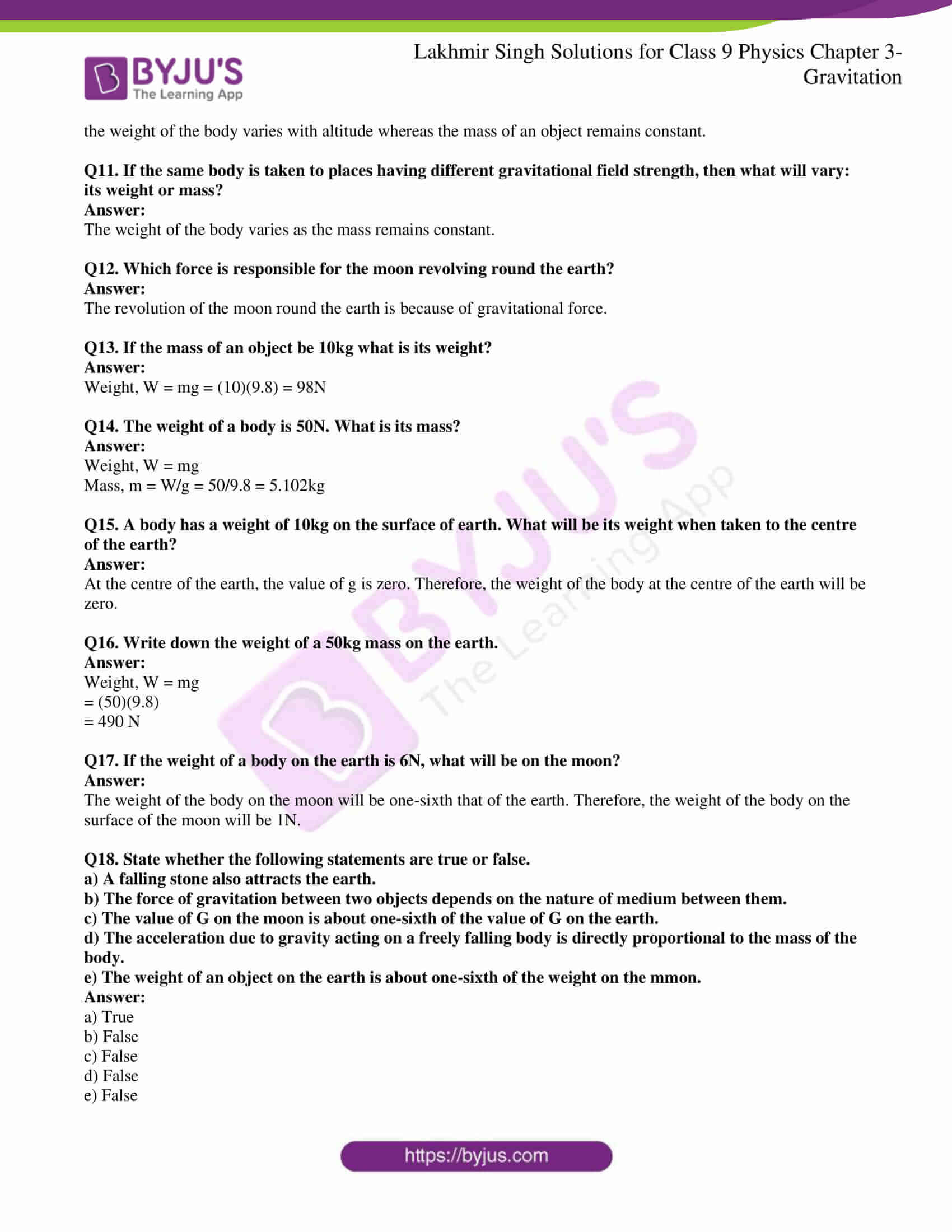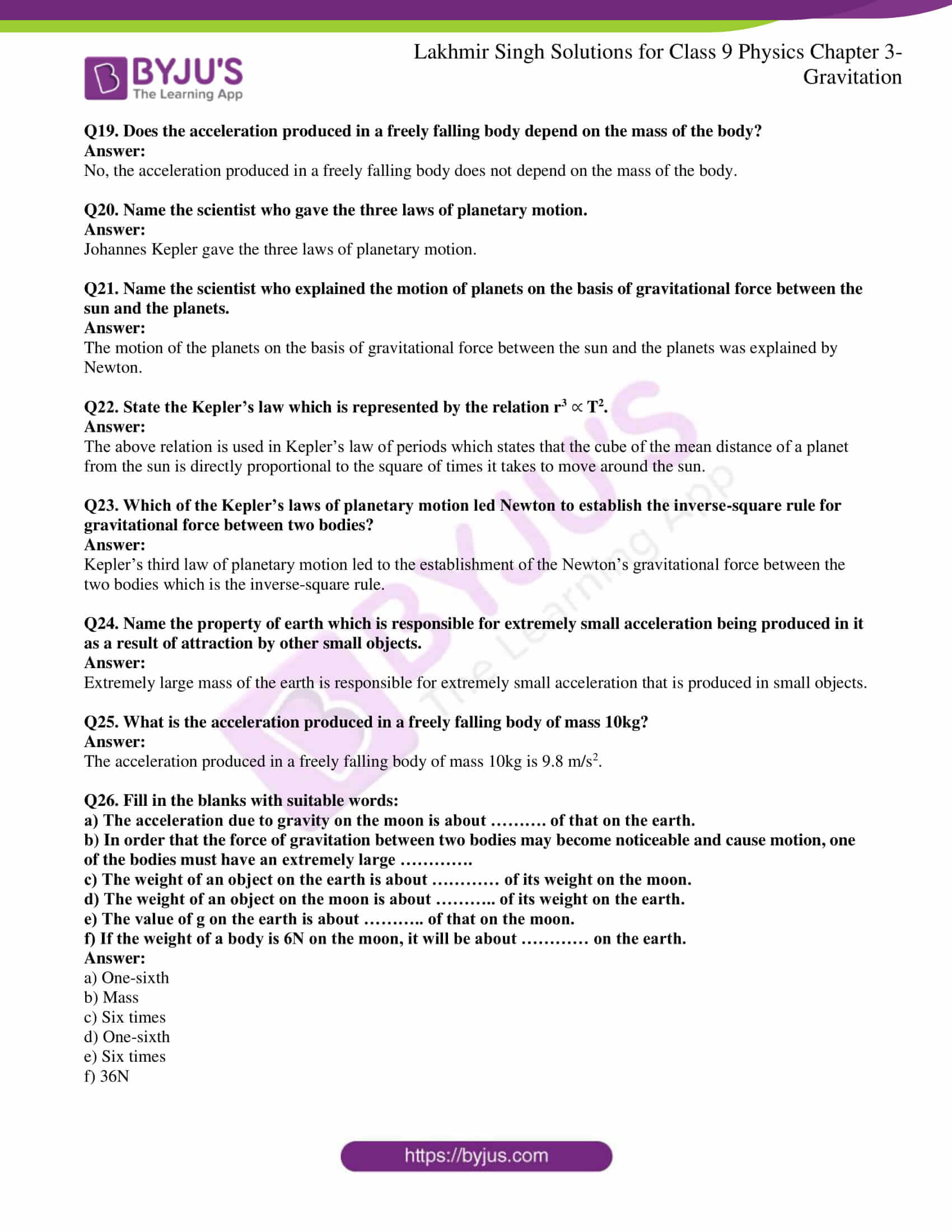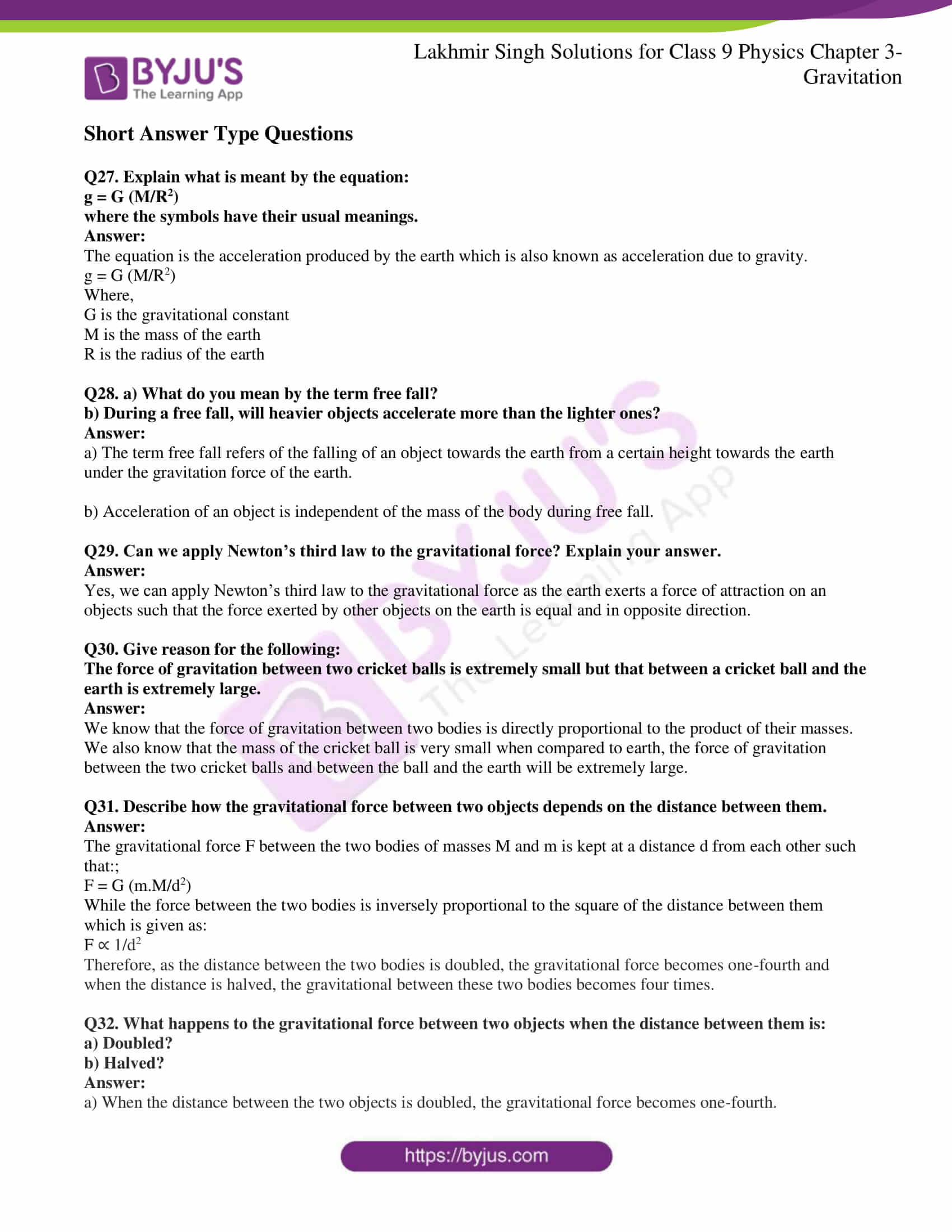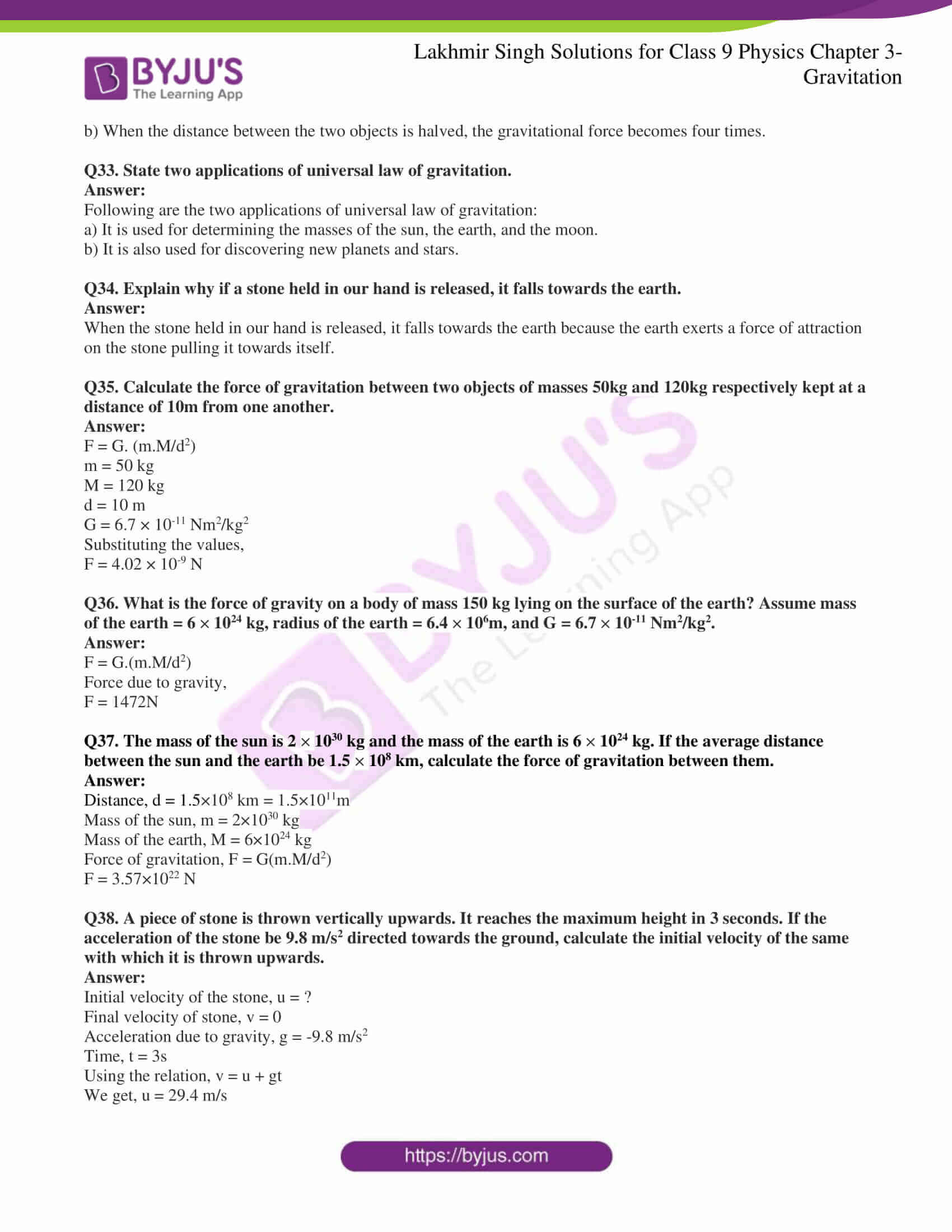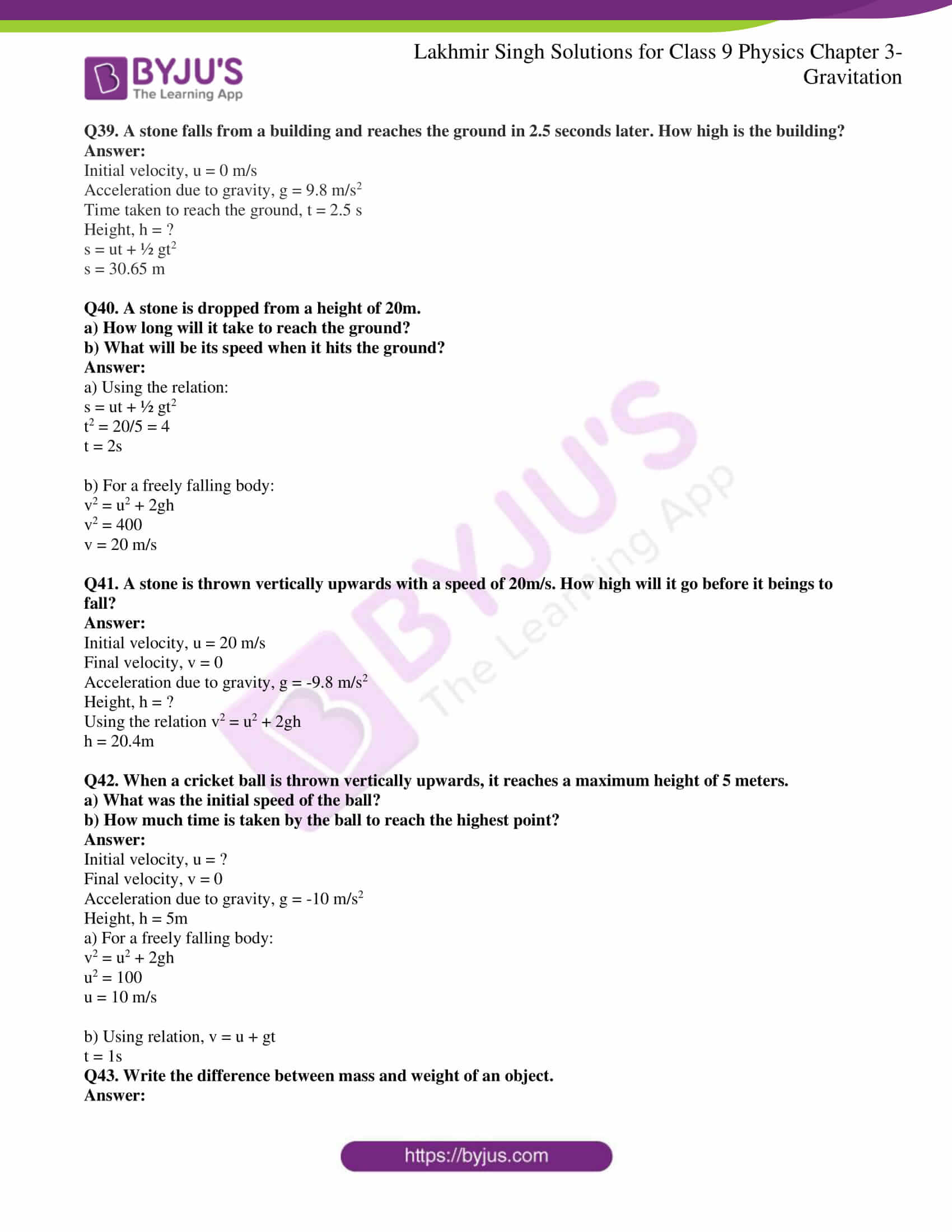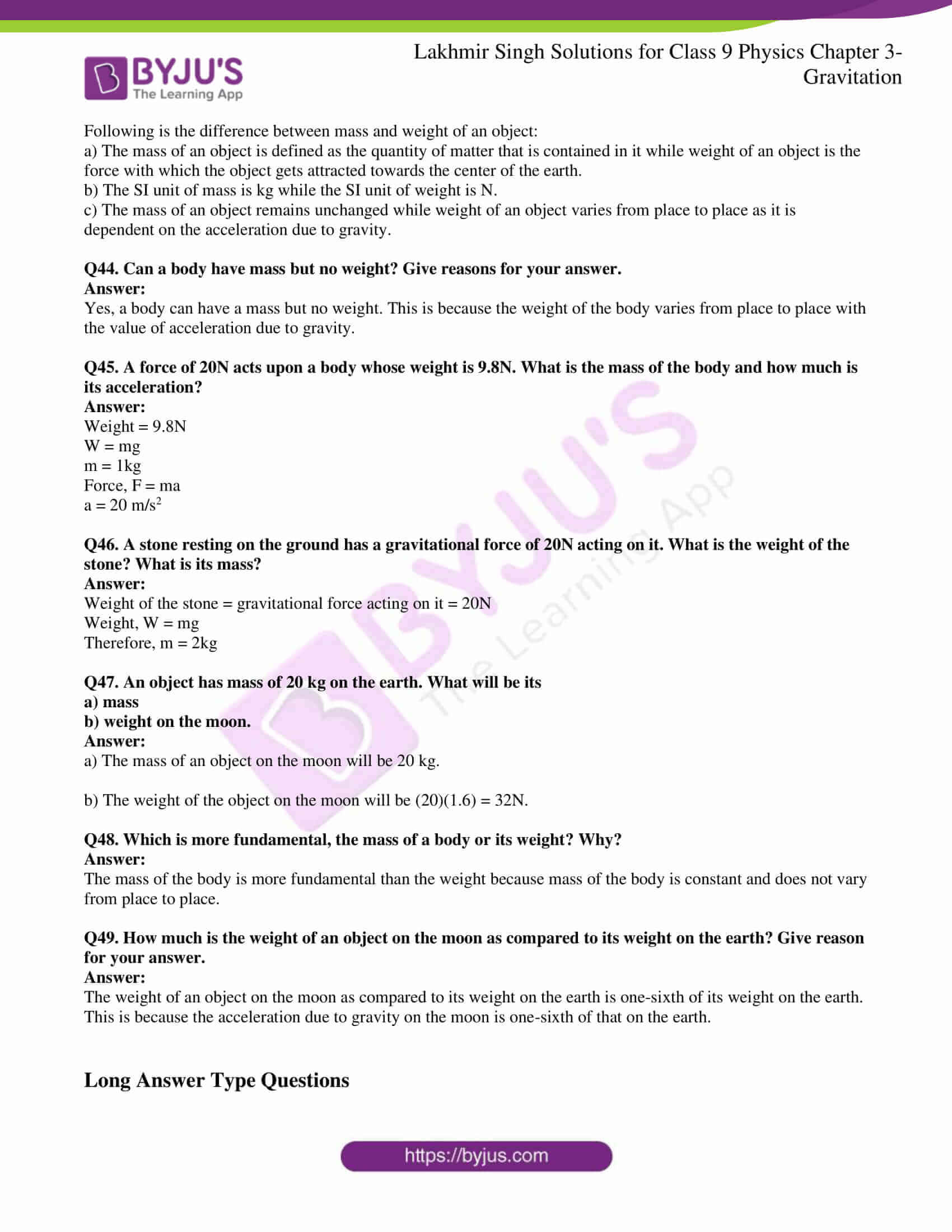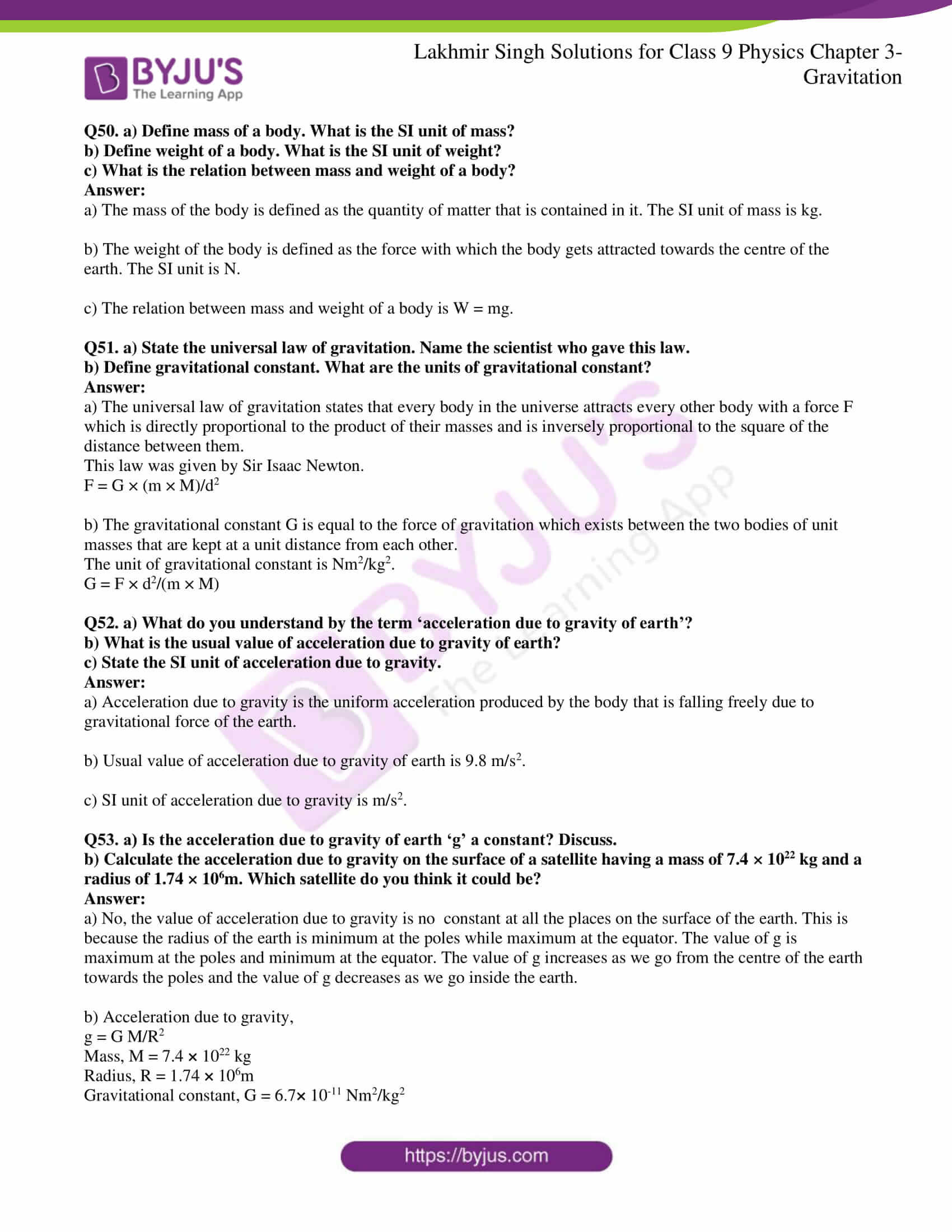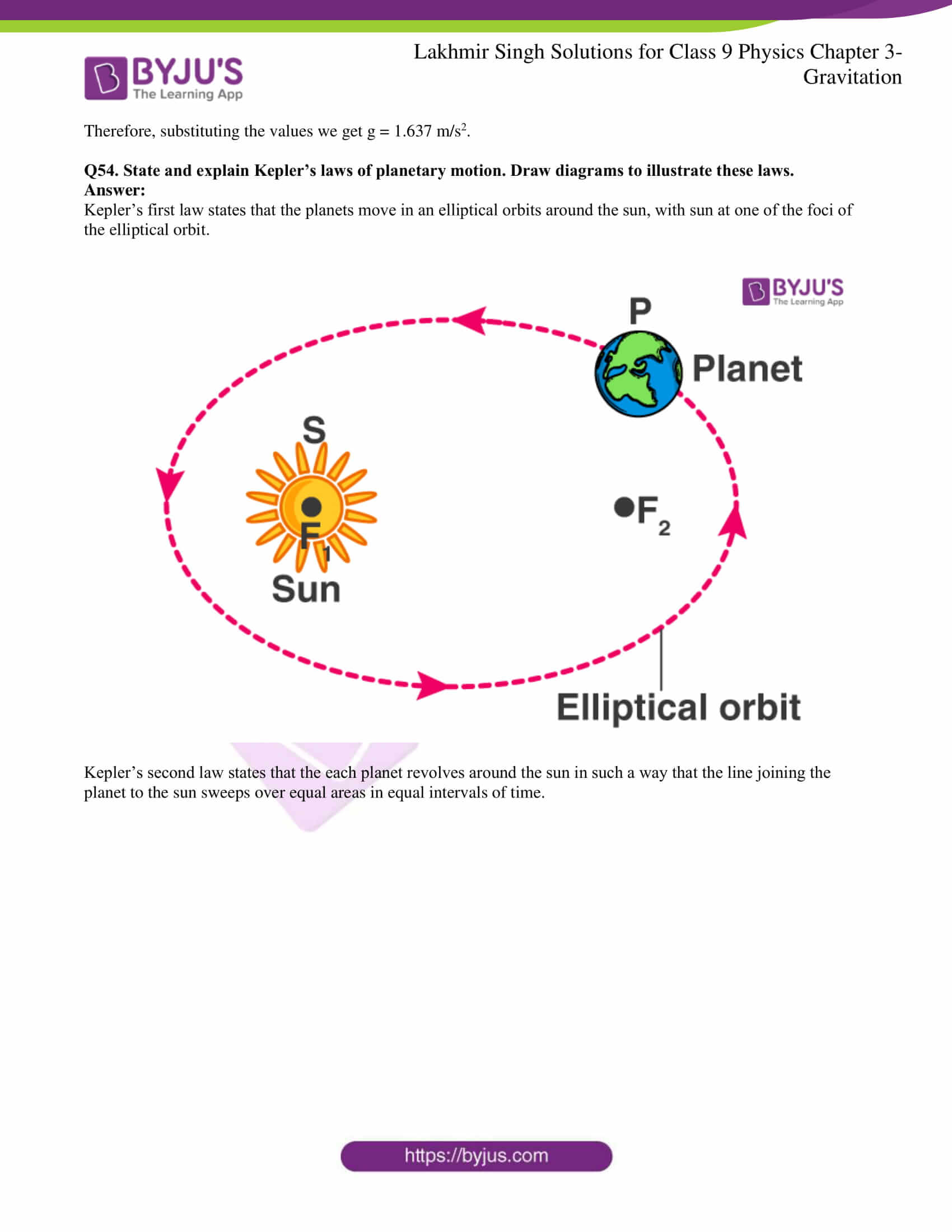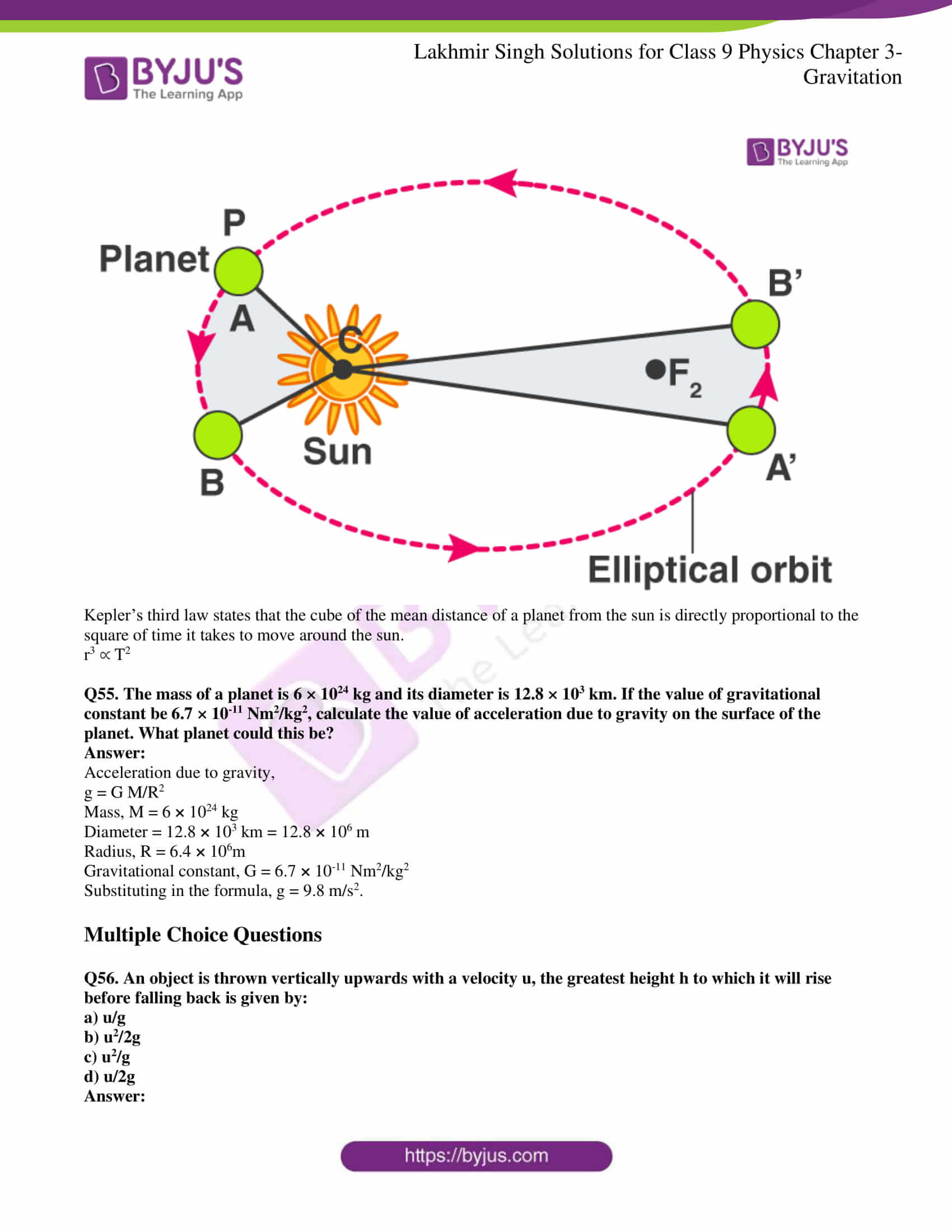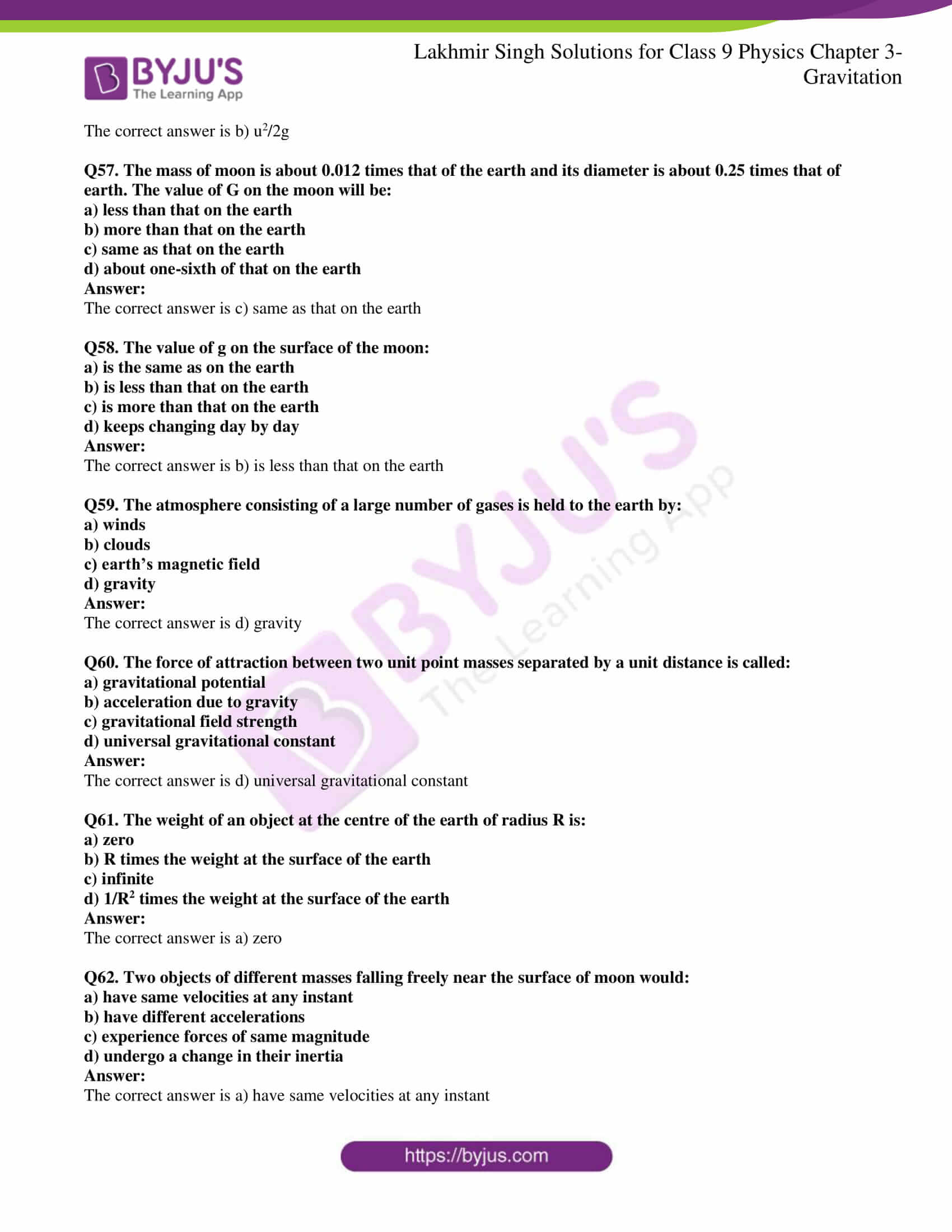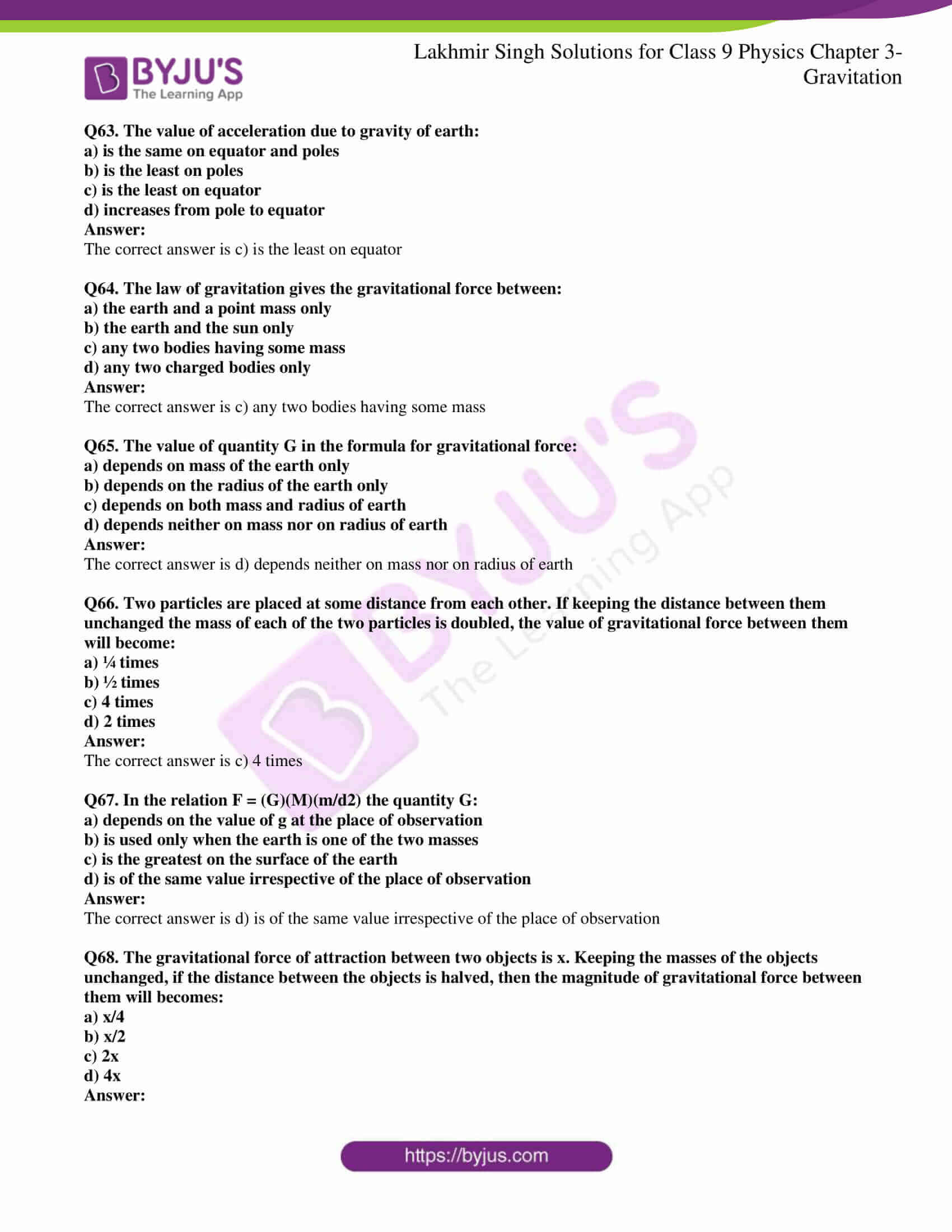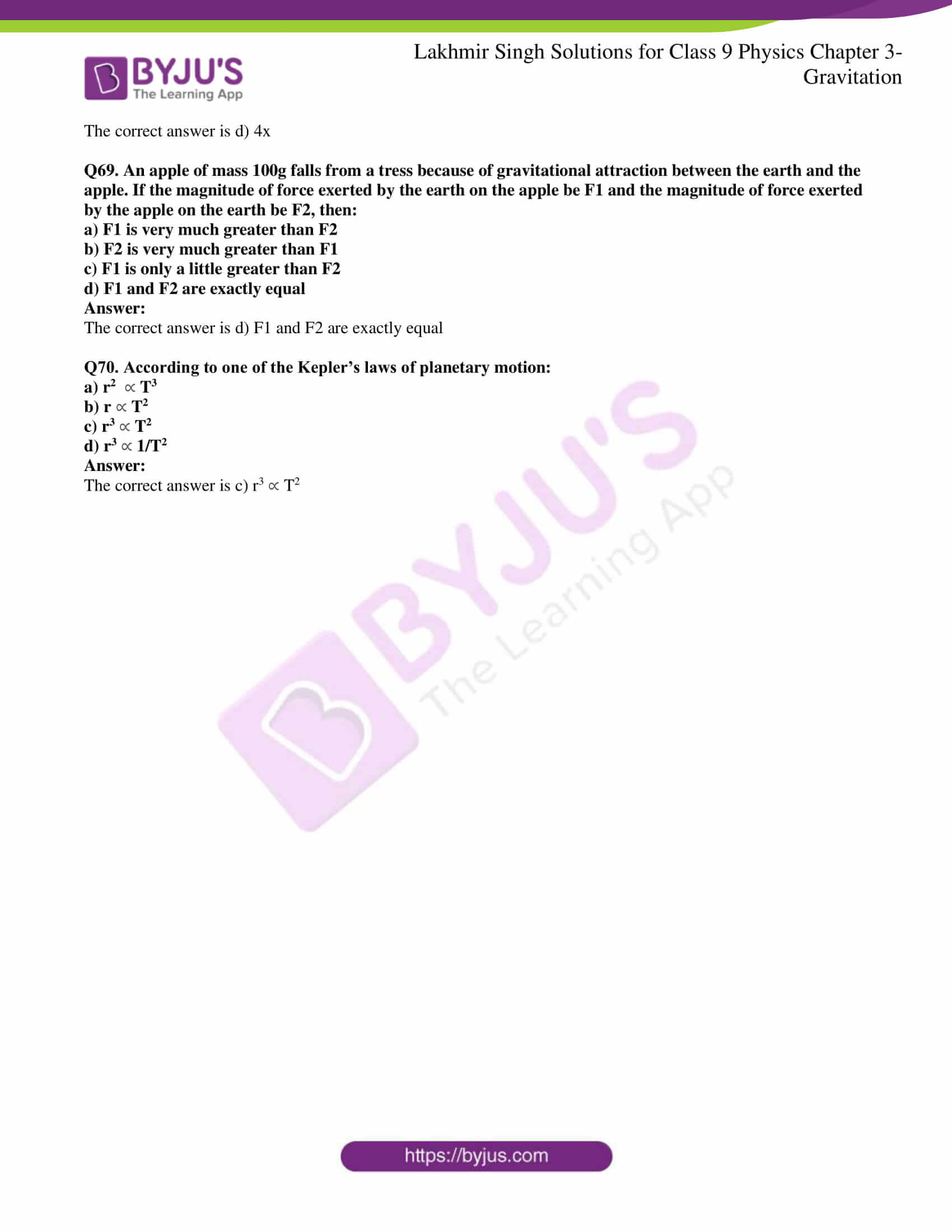Page No: 100

### Very Short Answer Type Questions

Q1. What is the value of gravitational constant G

a) on the earth

b) on the moon

The value of gravitational constant G on the earth as well as on the moon = 6.67× 10-11 Nm2/kg2.

The value of G always remains constant irrespective of the location.

Q2. When an object is dropped from a height, it accelerates and falls down. Name the force which accelerates the object.

The force that accelerates the object is gravitational force of the earth.

Q3. Give the formula for the gravitational force F between two bodies of masses M and m kept at a distance d from each other.

The gravitational force F between two bodies of masses M and m kept at a distance d from each other is given as:

F = G × (m × M)/d2

Where G = 6.67× 10-11 Nm2/kg2

Q4. What force is responsible for the earth revolving round the sun?

Gravitational force is responsible for the earth revolving around the sun.

Q5. What name has been given to the force with which two objects lying apart attract each other?

Gravitational force attracts the two objects lying apart from each other.

Q6. What type of force is involved in the formation of tides in the sea?

The formation of tides in the sea is because of the gravitational force.

Q7. Which force is responsible for holding the solar system together?

Gravitational force is responsible for holding the solar system together.

Q8. What is the weight of a 1kg mass of the earth? When g = 9.8 m/s2.

Weight, w = mg = (1) (9.8) = 9.8N

Q9. On what factor/factors does the weight of a body depend?

The weight of a body depends on the mass and on the acceleration due to gravity which varies from place to place.

Q10. As the altitude of a body increases, do the weight and mass both vary?

the weight of the body varies with altitude whereas the mass of an object remains constant.

Q11. If the same body is taken to places having different gravitational field strength, then what will vary: its weight or mass?

The weight of the body varies as the mass remains constant.

Q12. Which force is responsible for the moon revolving round the earth?

The revolution of the moon round the earth is because of gravitational force.

Q13. If the mass of an object be 10kg what is its weight?

Weight, W = mg = (10)(9.8) = 98N

Q14. The weight of a body is 50N. What is its mass?

Weight, W = mg

Mass, m = W/g = 50/9.8 = 5.102kg

Q15. A body has a weight of 10kg on the surface of earth. What will be its weight when taken to the centre of the earth?

At the centre of the earth, the value of g is zero. Therefore, the weight of the body at the centre of the earth will be zero.

Q16. Write down the weight of a 50kg mass on the earth.

Weight, W = mg

= (50)(9.8)

= 490 N

Q17. If the weight of a body on the earth is 6N, what will be on the moon?

The weight of the body on the moon will be one-sixth that of the earth. Therefore, the weight of the body on the surface of the moon will be 1N.

Q18. State whether the following statements are true or false.

a) A falling stone also attracts the earth.

b) The force of gravitation between two objects depends on the nature of medium between them.

c) The value of G on the moon is about one-sixth of the value of G on the earth.

d) The acceleration due to gravity acting on a freely falling body is directly proportional to the mass of the body.

e) The weight of an object on the earth is about one-sixth of the weight on the mmon.

a) True

b) False

c) False

d) False

e) False

Q19. Does the acceleration produced in a freely falling body depend on the mass of the body?

No, the acceleration produced in a freely falling body does not depend on the mass of the body.

Q20. Name the scientist who gave the three laws of planetary motion.

Johannes Kepler gave the three laws of planetary motion.

Q21. Name the scientist who explained the motion of planets on the basis of gravitational force between the sun and the planets.

The motion of the planets on the basis of gravitational force between the sun and the planets was explained by Newton.

Q22. State the Kepler’s law which is represented by the relation r3 T2.

The above relation is used in Kepler’s law of periods which states that the cube of the mean distance of a planet from the sun is directly proportional to the square of times it takes to move around the sun.

Q23. Which of the Kepler’s laws of planetary motion led Newton to establish the inverse-square rule for gravitational force between two bodies?

Kepler’s third law of planetary motion led to the establishment of the Newton’s gravitational force between the two bodies which is the inverse-square rule.

Q24. Name the property of earth which is responsible for extremely small acceleration being produced in it as a result of attraction by other small objects.

Extremely large mass of the earth is responsible for extremely small acceleration that is produced in small objects.

Q25. What is the acceleration produced in a freely falling body of mass 10kg?

The acceleration produced in a freely falling body of mass 10kg is 9.8 m/s2.

Q26. Fill in the blanks with suitable words:

a) The acceleration due to gravity on the moon is about ………. of that on the earth.

b) In order that the force of gravitation between two bodies may become noticeable and cause motion, one of the bodies must have an extremely large ………….

c) The weight of an object on the earth is about ………… of its weight on the moon.

d) The weight of an object on the moon is about ……….. of its weight on the earth.

e) The value of g on the earth is about ……….. of that on the moon.

f) If the weight of a body is 6N on the moon, it will be about ………… on the earth.

a) One-sixth

b) Mass

c) Six times

d) One-sixth

e) Six times

f) 36N

Q27. Explain what is meant by the equation:

g = G (M/R2)

where the symbols have their usual meanings.

The equation is the acceleration produced by the earth which is also known as acceleration due to gravity.

g = G (M/R2)

Where,

G is the gravitational constant

M is the mass of the earth

R is the radius of the earth

Q28. a) What do you mean by the term free fall?

b) During a free fall, will heavier objects accelerate more than the lighter ones?

a) The term free fall refers of the falling of an object towards the earth from a certain height towards the earth under the gravitation force of the earth.

b) Acceleration of an object is independent of the mass of the body during free fall.

Q29. Can we apply Newton’s third law to the gravitational force? Explain your answer.

Yes, we can apply Newton’s third law to the gravitational force as the earth exerts a force of attraction on an objects such that the force exerted by other objects on the earth is equal and in opposite direction.

Q30. Give reason for the following:

The force of gravitation between two cricket balls is extremely small but that between a cricket ball and the earth is extremely large.

We know that the force of gravitation between two bodies is directly proportional to the product of their masses. We also know that the mass of the cricket ball is very small when compared to earth, the force of gravitation between the two cricket balls and between the ball and the earth will be extremely large.

Q31. Describe how the gravitational force between two objects depends on the distance between them.

The gravitational force F between the two bodies of masses M and m is kept at a distance d from each other such that:;

F = G (m.M/d2)

While the force between the two bodies is inversely proportional to the square of the distance between them which is given as:

F ∝ 1/d2

Therefore, as the distance between the two bodies is doubled, the gravitational force becomes one-fourth and when the distance is halved, the gravitational between these two bodies becomes four times.

Q32. What happens to the gravitational force between two objects when the distance between them is:

a) Doubled?

b) Halved?

a) When the distance between the two objects is doubled, the gravitational force becomes one-fourth.

b) When the distance between the two objects is halved, the gravitational force becomes four times.

Q33. State two applications of universal law of gravitation.

Following are the two applications of universal law of gravitation:

a) It is used for determining the masses of the sun, the earth, and the moon.

b) It is also used for discovering new planets and stars.

Q34. Explain why if a stone held in our hand is released, it falls towards the earth.

When the stone held in our hand is released, it falls towards the earth because the earth exerts a force of attraction on the stone pulling it towards itself.

Q35. Calculate the force of gravitation between two objects of masses 50kg and 120kg respectively kept at a distance of 10m from one another.

F = G. (m.M/d2)

m = 50 kg

M = 120 kg

d = 10 m

G = 6.7 × 10-11 Nm2/kg2

Substituting the values,

F = 4.02 × 10-9 N

Q36. What is the force of gravity on a body of mass 150 kg lying on the surface of the earth? Assume mass of the earth = 6 × 1024 kg, radius of the earth = 6.4 × 106m, and G = 6.7 × 10-11 Nm2/kg2.

F = G.(m.M/d2)

Force due to gravity,

F = 1472N

Q37. The mass of the sun is 2 × 1030 kg and the mass of the earth is 6 × 1024 kg. If the average distance between the sun and the earth be 1.5 × 108 km, calculate the force of gravitation between them.

Distance, d = 1.5×108 km = 1.5×1011m

Mass of the sun, m = 2×1030 kg

Mass of the earth, M = 6×1024 kg

Force of gravitation, F = G(m.M/d2)

F = 3.57×1022 N

Q38. A piece of stone is thrown vertically upwards. It reaches the maximum height in 3 seconds. If the acceleration of the stone be 9.8 m/s2 directed towards the ground, calculate the initial velocity of the same with which it is thrown upwards.

Initial velocity of the stone, u = ?

Final velocity of stone, v = 0

Acceleration due to gravity, g = -9.8 m/s2

Time, t = 3s

Using the relation, v = u + gt

We get, u = 29.4 m/s

Q39. A stone falls from a building and reaches the ground in 2.5 seconds later. How high is the building?

Initial velocity, u = 0 m/s

Acceleration due to gravity, g = 9.8 m/s2

Time taken to reach the ground, t = 2.5 s

Height, h = ?

s = ut + ½ gt2

s = 30.65 m

Q40. A stone is dropped from a height of 20m.

a) How long will it take to reach the ground?

b) What will be its speed when it hits the ground?

a) Using the relation:

s = ut + ½ gt2

t2 = 20/5 = 4

t = 2s

b) For a freely falling body:

v2 = u2 + 2gh

v2 = 400

v = 20 m/s

Q41. A stone is thrown vertically upwards with a speed of 20m/s. How high will it go before it beings to fall?

Initial velocity, u = 20 m/s

Final velocity, v = 0

Acceleration due to gravity, g = -9.8 m/s2

Height, h = ?

Using the relation v2 = u2 + 2gh

h = 20.4m

Q42. When a cricket ball is thrown vertically upwards, it reaches a maximum height of 5 meters.

a) What was the initial speed of the ball?

b) How much time is taken by the ball to reach the highest point?

Initial velocity, u = ?

Final velocity, v = 0

Acceleration due to gravity, g = -10 m/s2

Height, h = 5m

a) For a freely falling body:

v2 = u2 + 2gh

u2 = 100

u = 10 m/s

b) Using relation, v = u + gt

t = 1s

Q43. Write the difference between mass and weight of an object.

Following is the difference between mass and weight of an object:

a) The mass of an object is defined as the quantity of matter that is contained in it while weight of an object is the force with which the object gets attracted towards the center of the earth.

b) The SI unit of mass is kg while the SI unit of weight is N.

c) The mass of an object remains unchanged while weight of an object varies from place to place as it is dependent on the acceleration due to gravity.

Q44. Can a body have mass but no weight? Give reasons for your answer.

Yes, a body can have a mass but no weight. This is because the weight of the body varies from place to place with the value of acceleration due to gravity.

Q45. A force of 20N acts upon a body whose weight is 9.8N. What is the mass of the body and how much is its acceleration?

Weight = 9.8N

W = mg

m = 1kg

Force, F = ma

a = 20 m/s2

Q46. A stone resting on the ground has a gravitational force of 20N acting on it. What is the weight of the stone? What is its mass?

Weight of the stone = gravitational force acting on it = 20N

Weight, W = mg

Therefore, m = 2kg

Q47. An object has mass of 20 kg on the earth. What will be its

a) mass

b) weight on the moon.

a) The mass of an object on the moon will be 20 kg.

b) The weight of the object on the moon will be (20)(1.6) = 32N.

Q48. Which is more fundamental, the mass of a body or its weight? Why?

The mass of the body is more fundamental than the weight because mass of the body is constant and does not vary from place to place.

Q49. How much is the weight of an object on the moon as compared to its weight on the earth? Give reason for your answer.

The weight of an object on the moon as compared to its weight on the earth is one-sixth of its weight on the earth. This is because the acceleration due to gravity on the moon is one-sixth of that on the earth.

Q50. a) Define mass of a body. What is the SI unit of mass?

b) Define weight of a body. What is the SI unit of weight?

c) What is the relation between mass and weight of a body?

a) The mass of the body is defined as the quantity of matter that is contained in it. The SI unit of mass is kg.

b) The weight of the body is defined as the force with which the body gets attracted towards the centre of the earth. The SI unit is N.

c) The relation between mass and weight of a body is W = mg.

Q51. a) State the universal law of gravitation. Name the scientist who gave this law.

b) Define gravitational constant. What are the units of gravitational constant?

a) The universal law of gravitation states that every body in the universe attracts every other body with a force F which is directly proportional to the product of their masses and is inversely proportional to the square of the distance between them.

This law was given by Sir Isaac Newton.

F = G × (m × M)/d2

b) The gravitational constant G is equal to the force of gravitation which exists between the two bodies of unit masses that are kept at a unit distance from each other.

The unit of gravitational constant is Nm2/kg2.

G = F × d2/(m × M)

Q52. a) What do you understand by the term ‘acceleration due to gravity of earth’?

b) What is the usual value of acceleration due to gravity of earth?

c) State the SI unit of acceleration due to gravity.

a) Acceleration due to gravity is the uniform acceleration produced by the body that is falling freely due to gravitational force of the earth.

b) Usual value of acceleration due to gravity of earth is 9.8 m/s2.

c) SI unit of acceleration due to gravity is m/s2.

Q53. a) Is the acceleration due to gravity of earth ‘g’ a constant? Discuss.

b) Calculate the acceleration due to gravity on the surface of a satellite having a mass of 7.4 × 1022 kg and a radius of 1.74 × 106m. Which satellite do you think it could be?

a) No, the value of acceleration due to gravity is no constant at all the places on the surface of the earth. This is because the radius of the earth is minimum at the poles while maximum at the equator. The value of g is maximum at the poles and minimum at the equator. The value of g increases as we go from the centre of the earth towards the poles and the value of g decreases as we go inside the earth.

b) Acceleration due to gravity,

g = G M/R2

Mass, M = 7.4 × 1022 kg

Radius, R = 1.74 × 106m

Gravitational constant, G = 6.7× 10-11 Nm2/kg2

Therefore, substituting the values we get g = 1.637 m/s2.

Q54. State and explain Kepler’s laws of planetary motion. Draw diagrams to illustrate these laws.

Kepler’s first law states that the planets move in an elliptical orbits around the sun, with sun at one of the foci of the elliptical orbit.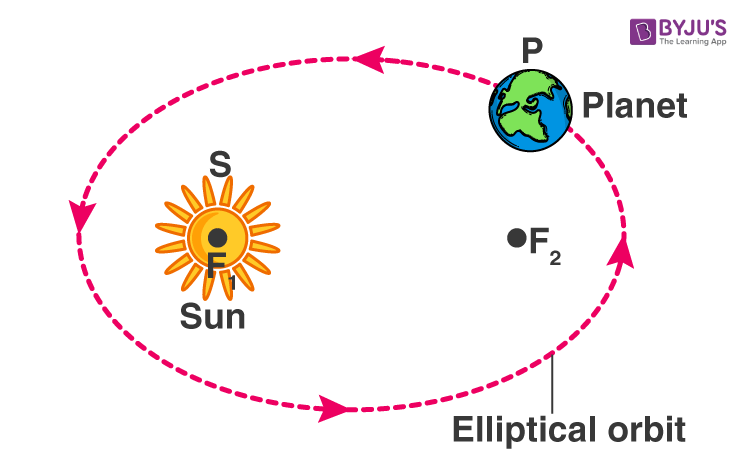Kepler’s second law states that the each planet revolves around the sun in such a way that the line joining the planet to the sun sweeps over equal areas in equal intervals of time.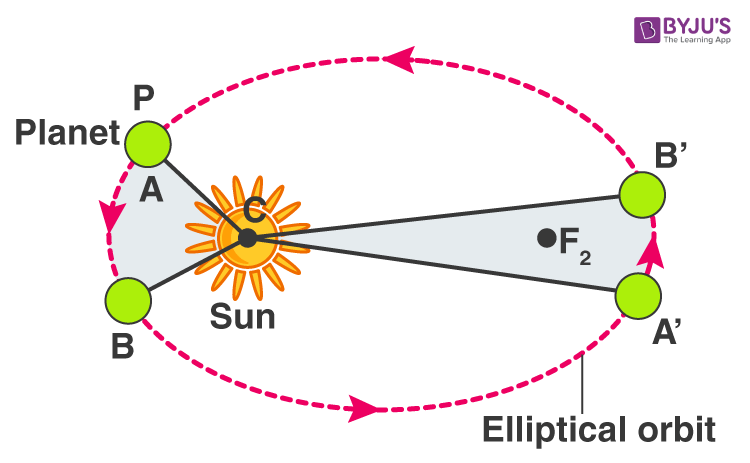Kepler’s third law states that the cube of the mean distance of a planet from the sun is directly proportional to the square of time it takes to move around the sun.

r3 ∝ T2

Q55. The mass of a planet is 6 × 1024 kg and its diameter is 12.8 × 103 km. If the value of gravitational constant be 6.7 × 10-11 Nm2/kg2, calculate the value of acceleration due to gravity on the surface of the planet. What planet could this be?

Acceleration due to gravity,

g = G M/R2

Mass, M = 6 × 1024 kg

Diameter = 12.8 × 103 km = 12.8 × 106 m

Radius, R = 6.4 × 106m

Gravitational constant, G = 6.7 × 10-11 Nm2/kg2

Substituting in the formula, g = 9.8 m/s2.

### Multiple Choice Questions

Q56. An object is thrown vertically upwards with a velocity u, the greatest height h to which it will rise before falling back is given by:

a) u/g

b) u2/2g

c) u2/g

d) u/2g

The correct answer is b) u2/2g

Q57. The mass of moon is about 0.012 times that of the earth and its diameter is about 0.25 times that of earth. The value of G on the moon will be:

a) less than that on the earth

b) more than that on the earth

c) same as that on the earth

d) about one-sixth of that on the earth

The correct answer is c) same as that on the earth

Q58. The value of g on the surface of the moon:

a) is the same as on the earth

b) is less than that on the earth

c) is more than that on the earth

d) keeps changing day by day

The correct answer is b) is less than that on the earth

Q59. The atmosphere consisting of a large number of gases is held to the earth by:

a) winds

b) clouds

c) earth’s magnetic field

d) gravity

The correct answer is d) gravity

Q60. The force of attraction between two unit point masses separated by a unit distance is called:

a) gravitational potential

b) acceleration due to gravity

c) gravitational field strength

d) universal gravitational constant

The correct answer is d) universal gravitational constant

Q61. The weight of an object at the centre of the earth of radius R is:

a) zero

b) R times the weight at the surface of the earth

c) infinite

d) 1/R2 times the weight at the surface of the earth

The correct answer is a) zero

Q62. Two objects of different masses falling freely near the surface of moon would:

a) have same velocities at any instant

b) have different accelerations

c) experience forces of same magnitude

d) undergo a change in their inertia

The correct answer is a) have same velocities at any instant

Q63. The value of acceleration due to gravity of earth:

a) is the same on equator and poles

b) is the least on poles

c) is the least on equator

d) increases from pole to equator

The correct answer is c) is the least on equator

Q64. The law of gravitation gives the gravitational force between:

a) the earth and a point mass only

b) the earth and the sun only

c) any two bodies having some mass

d) any two charged bodies only

The correct answer is c) any two bodies having some mass

Q65. The value of quantity G in the formula for gravitational force:

a) depends on mass of the earth only

b) depends on the radius of the earth only

c) depends on both mass and radius of earth

d) depends neither on mass nor on radius of earth

The correct answer is d) depends neither on mass nor on radius of earth

Q66. Two particles are placed at some distance from each other. If keeping the distance between them unchanged the mass of each of the two particles is doubled, the value of gravitational force between them will become:

a) ¼ times

b) ½ times

c) 4 times

d) 2 times

The correct answer is c) 4 times

Q67. In the relation F = (G)(M)(m/d2) the quantity G:

a) depends on the value of g at the place of observation

b) is used only when the earth is one of the two masses

c) is the greatest on the surface of the earth

d) is of the same value irrespective of the place of observation

The correct answer is d) is of the same value irrespective of the place of observation

Q68. The gravitational force of attraction between two objects is x. Keeping the masses of the objects unchanged, if the distance between the objects is halved, then the magnitude of gravitational force between them will becomes:

a) x/4

b) x/2

c) 2x

d) 4x

The correct answer is d) 4x

Q69. An apple of mass 100g falls from a tress because of gravitational attraction between the earth and the apple. If the magnitude of force exerted by the earth on the apple be F1 and the magnitude of force exerted by the apple on the earth be F2, then:

a) F1 is very much greater than F2

b) F2 is very much greater than F1

c) F1 is only a little greater than F2

d) F1 and F2 are exactly equal

The correct answer is d) F1 and F2 are exactly equal

Q70. According to one of the Kepler’s laws of planetary motion:

a) r2 T3

b) r T2

c) r3 T2

d) r3 1/T2